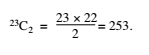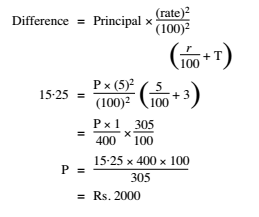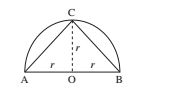# RRB ALP 2018 Practice Test Papers | Arithmetic Questions (Day-27)

Dear Aspirants, Here we have given the Important RRB ALP & Technicians Exam 2018 Practice Test Papers. Candidates those who are preparing for RRB ALP 2018 can practice these Arithmetic Questions to get more confidence to Crack RRB 2018 Examination.

[WpProQuiz 1940]

Click “Start Quiz” to attend these Questions and view Explanation

1. 5 pencils and 4 erasers cost Rs. 13 whereas 9 pencils and 5 erasers cost Rs. 19. Then the price of 6 pencils and 3 erasers is—

(A) Rs. 9

(B) Rs. 12

(C) Rs. 15

(D) Rs. 18

1. A two digit number is such that when it is divided by the largest single digit prime number leaves remainder 4. If the difference of the two digits is 4, then the number is—

(A) 73

(B) 25

(C) 53

(D) 95

1. The ratio of the present age of a man and his wife is 4: 3 and 4 years hence, the ratio of their ages will be 9: 7. If the ratio of their ages at the time of their marriage was 13: 9, how many years ago were they married?

(A) 4

(B) 8

(C) 6

(D) 9

1. 50 workers can complete a job in 6 days working 8 hours a day. If 40 workers are employed to complete the job in 20 days, then the number of hours they should be working per day is—

(A) 4 hours

(B) 6 hours

(C) 9 hours

(D) 3 hours

1. The number of straight lines that can be drawn in a plane with 23 given points, assuming that no three of them are collinear is—

(A) 253

(B) 46

(C) 2·23!

(D) 21!

1. If the difference between the compound interest and simple interest on a certain sum at 5% per annum for 3 years is Rs. 15·25, what is the sum?

(A) Rs. 2000

(B) Rs. 1000

(C) Rs. 2500

(D) Rs. 1500

1. In a group there are 25 men and 20 women. Average weight of a man is 48 kg while the average weight of a woman is 30 kg. What is the average weight of the group?

(A) 35 kg

(B) 39 kg

(C) 40 kg

(D) 45 kg

1. A sum of money was distributed among 120 men and some ladies in the ratio of 15: 21. If each man got Rs. 5 and a lady Rs. 4. What is the total number of ladies?

(A) 220

(B) 200

(C) 190

(D) 210

1. A train 130 metres, running at a speed of 45 km/hr, crosses a bridge in 30 seconds. What is the length of the bridge?

(A) 200 m

(B) 225 m

(C) 245 m

(D) 250 m

1. Area of the largest triangle drawn in a semicircle of radius r will be—

(A) r2

(B) r3

(C) 2r2

(D) 2r3

Let the price of pencil be Rs. x and the cost of erasers be Rs. y.

5x + 4y = 13 …(i)

9x + 5y = 19 …(ii)

By taking together (i) and (ii) and calculating x = Rs. 1

Placing the value of ‘x’ in equation (i)

5 + 4y = 13 y = Rs. 2

then the price of 6 pencils and 3 erasers is 6 × 1 + 3 × 2= Rs. 12

Largest single digit prime number=7

95%7=4

Let the age of man be 4x and his wife 3x, after four years

4x + 4/ 3x + 4 = 9 /7

28x + 28 = 27x + 36

x = 36 – 28 = 8

then the age of man of 8 × 4 = 32 years

the age of his wife = 8 × 3 = 24 years

Let the years be t

32 – t/24 – t = 13 /9

288 – 9t = 312 – 13t

4t = 24

t = 24 /4 = 6 years

x = 50 × 6 × 8 /40 × 20 = 3 hourstotal weight=25×48+20×30=1800

Avg weight= 1800/(20+25)=40

Let the number of women be x 1

20 × 5/( x × 4) = 15/ 21

120 × 5 × 21 = 15 × x × 4

∴ x = 120 × 5 × 21 /(15 × 4) = 210

Let Length of bridge be x

45×5/18=(130+x)/30

X=245

Perpendicular height of the largest triangle drawn in a semi-circle of radius r = r∴ Area of the largest possible triangle = 1/ 2 (base × height) = 1/ 2 (2r) × (r) = r2

RRB ALP 2018 Practice Test Papers | Arithmetic Questions (Day-1)

RRB ALP 2018 Practice Test Papers | Arithmetic Questions (Day-2)

RRB ALP 2018 Practice Test Papers | Arithmetic Questions (Day-3)

RRB ALP 2018 Practice Test Papers | Arithmetic Questions (Day-4)

RRB ALP 2018 Practice Test Papers | Arithmetic Questions (Day-5)

RRB ALP 2018 Practice Test Papers | Arithmetic Questions (Day-6)

RRB ALP 2018 Practice Test Papers | Arithmetic Questions (Day-7)

RRB ALP 2018 Practice Test Papers | Arithmetic Questions (Day-8)

RRB ALP 2018 Practice Test Papers | Arithmetic Questions (Day-9)

RRB ALP 2018 Practice Test Papers | Arithmetic Questions (Day-10)

RRB ALP 2018 Practice Test Papers | Arithmetic Questions (Day-11)

RRB ALP 2018 Practice Test Papers | Arithmetic Questions (Day-12)

RRB ALP 2018 Practice Test Papers | Arithmetic Questions (Day-13)

RRB ALP 2018 Practice Test Papers | Arithmetic Questions (Day-14)

RRB ALP 2018 Practice Test Papers | Arithmetic Questions (Day-15)

RRB ALP 2018 Practice Test Papers | Arithmetic Questions (Day-16)

RRB ALP 2018 Practice Test Papers | Arithmetic Questions (Day-17)

RRB ALP 2018 Practice Test Papers | Arithmetic Questions (Day-18)

RRB ALP 2018 Practice Test Papers | Arithmetic Questions (Day-19)

RRB ALP 2018 Practice Test Papers | Arithmetic Questions (Day-20)

RRB ALP 2018 Practice Test Papers | Arithmetic Questions (Day-21)

RRB ALP 2018 Practice Test Papers | Arithmetic Questions (Day-22)

RRB ALP 2018 Practice Test Papers | Arithmetic Questions (Day-23)

RRB ALP 2018 Practice Test Papers | Arithmetic Questions (Day-24)

RRB ALP 2018 Practice Test Papers | Arithmetic Questions (Day-25)

RRB ALP 2018 Practice Test Papers | Arithmetic Questions (Day-26)